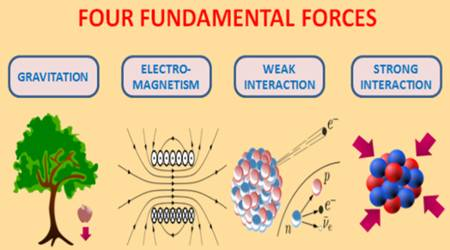# Strength of the Fundamental Forces

The strength of the Fundamental Forces

Among the four fundamental forces, strongest force is the strong nuclear force and weakest one is the gravitational force. The range of both strong and weak nuclear forces are short, whereas the range of gravitational and electromagnets forces are almost infinity.

Four types of fundamental forces are there in nature. They are –

• Gravitational force; weakest force; but an infinite range.
• Electromagnetic force; stronger, with infinite range.
• Strong nuclear force, strongest; but short range.
• Weak nuclear force; next weakest; but short range.The electromagnetic force causes electric and magnetic effects such as the repulsion between like electrical charges or the interaction of bar magnets. It is long-ranged but much weaker than the strong force. It can be attractive or repulsive and acts only between pieces of matter carrying an electrical charge. The weak force is responsible for radioactive decay and neutrino interactions. It has a very short range and, as its name indicates, it is very weak.

For comparison, if we take intensity or strength of strong nuclear force as 1, then intensities or strength of the weak nuclear force, electromagnetic force, and gravitational force are respectively 10-12, 10-2, and 10-39.